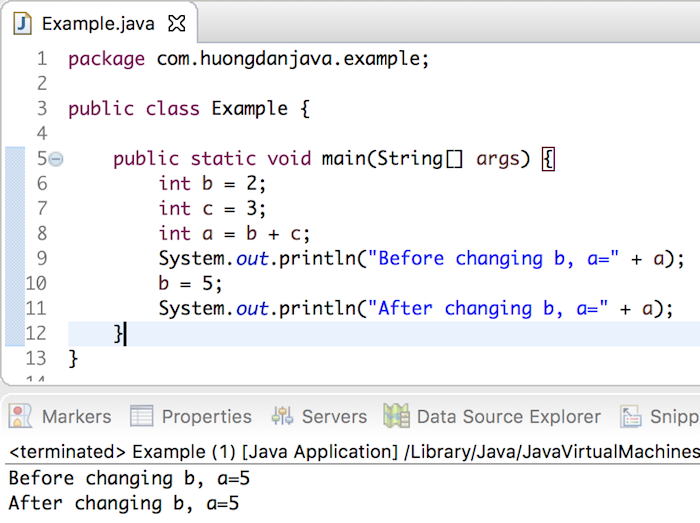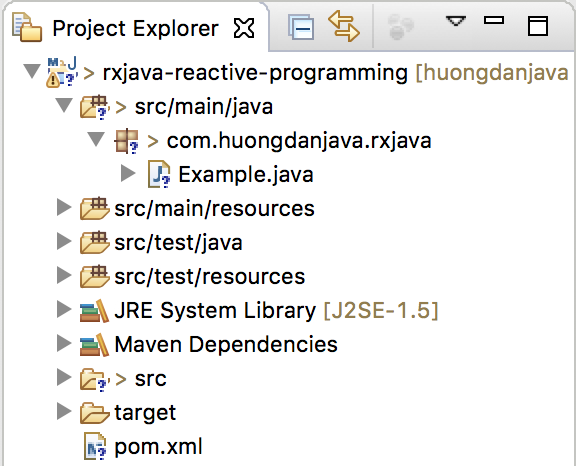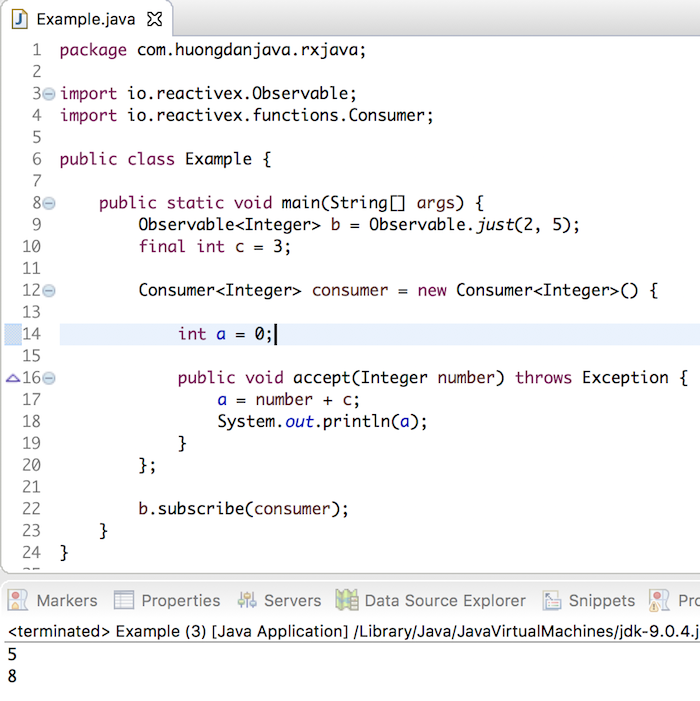# Introduction about Reactive Programming with RxJava

Reactive Programming is a programming model in which any change in the object that has other objects reference to, the objects reference therein recognize the change of the object.

One good example of this is that if you study Reactive Programming on the web, you will find:

If we have a variable as the sum of two numbers a variable b and a variable c, any change of the variable b and the variable c then will be a change in the value of the variable a.

In other programming models, although a is the sum of b and c, when we change b or c, the value of a does not change. Eg:

Result:With Reactive Programming, the value of a, after changing the value of b, will change. How about the change, let’s continue reading this tutorial. But first, I need to present you some concepts in Reactive Programming that you need to know.

• The first is the Publisher concept, which is subject to change of value.

In the example above, b or c is the Publisher object.

• The second is the concept of Subscriber, which is the object that will receive the change from the Publisher object.

In the above example, a is a Subscriber object.

• And the last concept is that Subscribe, which is the connection between Publisher and Subscriber, to Publisher can announce the change to the Subscriber.

To illustrate the Reactive Programming model, we will rewrite the example and use the RxJava library (Reactive Extensions for the JVM). RxJava is a library for deploying the Reactive Programming programming model in Java with full functionality that enables programmers to easily deploy Reactive Programming.

But first, I’m going to create a new Maven project with RxJava dependency:With RxJava dependency as follows:

In the RxJava library, the Observable or Flowable object represents a Publisher, while the Consumer or Subscriber object represents a Subscriber.

In the example above, to create a Publisher, you can declare the following:

With this declaration, the Observable object will create two values of 2 and 5 and these values will be updated for the Subscriber.

To create a Subscriber object, we will use the Consumer object as follows:

And to connect the Publisher and Subscriber above, we will use the subscribe() method of the Observable object:

At runtime, for each value that the Publisher generates, the Subscriber receives that value and updates to the variable a.

Here is the full code of the program:

Result: